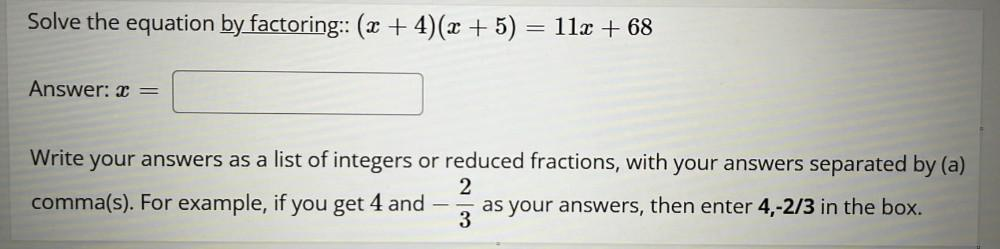Question:

# Solve the equation by factoring: (x + 4)(x + 5) = 11x + 68 x= Write your answers as a list of integers or reduced fractions, with your answers separated by (a) comma(s). For example, if you get 4 and-Solve the equation by factoring: (x + 4)(x + 5) = 11x + 68 x= Write your answers as a list of integers or reduced fractions, with your answers separated by (a) comma(s). For example, if you get 4 and-2/3 as your answers, then enter 4,-2/3 in the box.